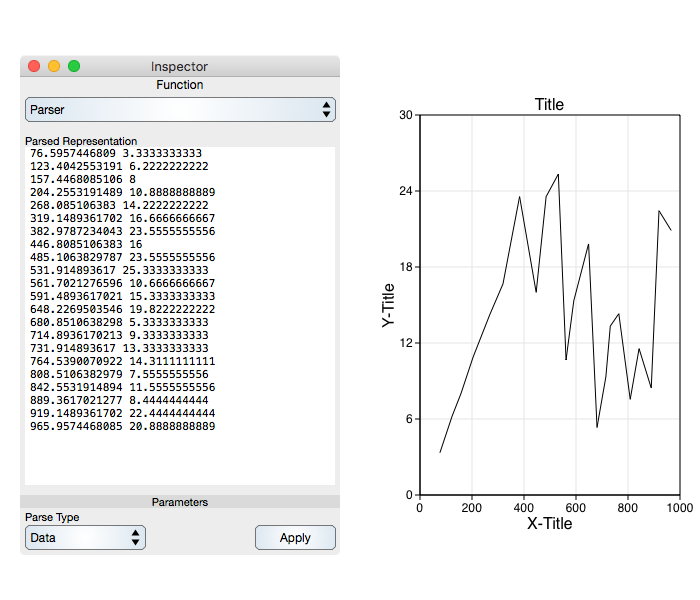Graph IDEExport And Import ► Raw Data Points

You can enter data points on a graphic-by-graphic (curve-by-curve) basis. To do that first make a prototype Data Graphic or other graphic that has points as a constitutive parameter. Then use that graphic's inspector editor Table interface or the Parser Inspector Editor to enter points.

The following shows how to enter data from a simple text representation using the data parser.

Point Data

Point data-oriented graphics include the Polygon, Function and Scatter graphics. Data points are entered as x and y whitespace separated pairs so, for example:

```5 6
6 20
7 40
```

Defines a curve with x-values 5, 6, 7 and y-values of 6, 20, 40 respectively.

Spline Data

Spline data-oriented graphics include the Cubic Bezier and Trajectory graphics. In the case of spline-oriented graphics you enter the beginning tangent end point, the actual data point and then the ending tangent end point in that order and all white space delimited. So, something like this:

```5 6 5 6 5 6
6 20 6 20 6 20
7 40 7 40 7 40
```

That case is graphically equivalent to the Point Data example of before because the tangent lines have zero length (coincide with the respective data point).

The Parser sub-inspector-editor has a Data representation interface where you can type in values of your data, or paste in all the values. An example is shown in the figure below. After typing or pasting the data click the Apply button to apply it to the respective data graphic, in this case a Function.